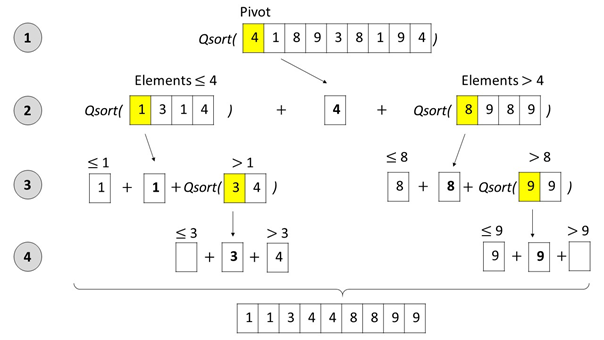# The Shortest Quicksort Algorithm in Python

5/5 - (1 vote)

Quicksort is not only a popular question in many code interviews – asked by Google, Facebook, and Amazon – but also a practical sorting algorithm that is fast, concise, and readable. Because of its beauty, you won’t find many introductions to algorithms that don’t discuss the Quicksort algorithm.

In this one-liner tutorial, you’ll learn about the popular sorting algorithm Quicksort. Surprisingly, a single line of Python code is all you need to write the Quicksort algorithm!

As you read the short article, feel free to play the following explainer video where I’ll guide you through the code:

Now, let’s go into the Quicksort algorithm!

## Quick Long Version

If you’re just looking for the code right away, I suggest you copy&paste the following code—a concise and efficient Quicksort implementation without all the fluff:

```def quicksort(my_list):
# recursion base case - empty list
if not my_list:
return []

# first element is pivot
pivot = my_list

# break up problem
smaller = [x for x in my_list[1:] if x < pivot]
greater = [x for x in my_list[1:] if x >= pivot]

# recursively solve problem and recombine solutions
return quicksort(smaller) + [pivot] + quicksort(greater)

print(quicksort([4, 4, 3, 2, 1, 8, 9]))
# [1, 2, 3, 4, 4, 8, 9]

print(quicksort(['Alice', 'Carl', 'Bob']))
# ['Alice', 'Bob', 'Carl']```

The remaining article will dive into the code and provide you some additional understanding of the algorithm.

## Quicksort Algorithm Idea

Quicksort sorts a list by recursively dividing the big problem (sorting one big list) into smaller problems (sorting two smaller lists) and combining the solutions from the smaller problems in a way that solves the big problem.

In order to solve each smaller problem, the same strategy is used recursively: the smaller problems are divided into even smaller subproblems, solved separately, and combined. Because of this strategy, Quicksort belongs to the class of “Divide and Conquer” algorithms.

The main idea of Quicksort is to select a pivot element and then place all elements that are greater than or equal to the pivot element to the right and all elements that are smaller than the pivot element to the left.

💡 Now, you have divided the big problem of sorting the list into two smaller subproblems: (1) sorting the right and (2) sorting the left partition of the list.

What you do now is to repeat this procedure recursively until you obtain a list with zero elements. This list is already sorted, so the recursion terminates.

The following Figure shows the Quicksort algorithm in action:Figure: The Quicksort algorithm selects a pivot element, splits up the list into (i) an unsorted sublist with all elements that are smaller or equal than the pivot, and (ii) an unsorted sublist with all elements that are larger than the pivot. Next, the Quicksort algorithm is called recursively on the two unsorted sublists to sort them. As soon as the sublists contain maximally one element, they are sorted by definition – the recursion ends. At every recursion level, the three sublists (left, pivot, right) are concatenated before the resulting list is handed to the higher recursion level.

Let’s dive into the code — a simple one-liner solution will do! 🙂

## Quicksort Python Code

Problem Formulation: Create a function `q` that implements the Quicksort algorithm in a single line of Python code by sorting and returning any argument specified as a list of integers.

Solution: The following one-liner solution for the Quicksort algorithm uses recursion to solve this problem.

```## The Data
unsorted = [33, 2, 3, 45, 6, 54, 33]

## The Quicksort One-Liner
q = lambda l: q([x for x in l[1:] if x <= l]) + [l] + q([x for x in l if x > l]) if l else []

## The Result
print(q(unsorted))```

## How It Works – Quicksort Code Explanation

We have already discussed the recursive Quicksort algorithm above. The one-liner resembles exactly the discussed algorithm. First, we create a new lambda function `q` which takes only one list argument `l`.

The lambda function has the following structure:

`lambda l: q(left) + pivot + q(right) if l else []`

The lambda function returns the empty list `[]` in the recursion base case (that is – the list to be sorted is empty and, therefore, trivially sorted).

In any other case, it selects the pivot element as the first element of list `l`, divides all elements into two sublists (`left` and `right`) based on whether they are smaller or larger than the pivot. To achieve this, we use simple list comprehension. You can watch our explainer video if you need a quick refresher:

As the two sublists are not necessarily sorted, we recursively execute the Quicksort algorithm on them. Finally, we combine all three lists and return the sorted list.

Therefore, the result is:

```## The Result
print(q(unsorted))
# [2, 3, 6, 33, 33, 45, 54]```

## Quicksort Instagram Summary

If you just want to get a quick idea of how to do it in more than one line, check out this Instagram post (swipe right):

## Where to Go From Here?

When a master coder looks at a snippet of source code such as the above ones, the meaning of the code pops into their head within seconds.

Have you reached this level of code understanding, yet? Do you want to reach it?

Solve a Python puzzle every day for continuous improvement. Over the years, you will reach this level of proficiency — even if you are doing this only on the side. Try the Finxter Premium Membership for free — it’ll guide you to Python mastery!